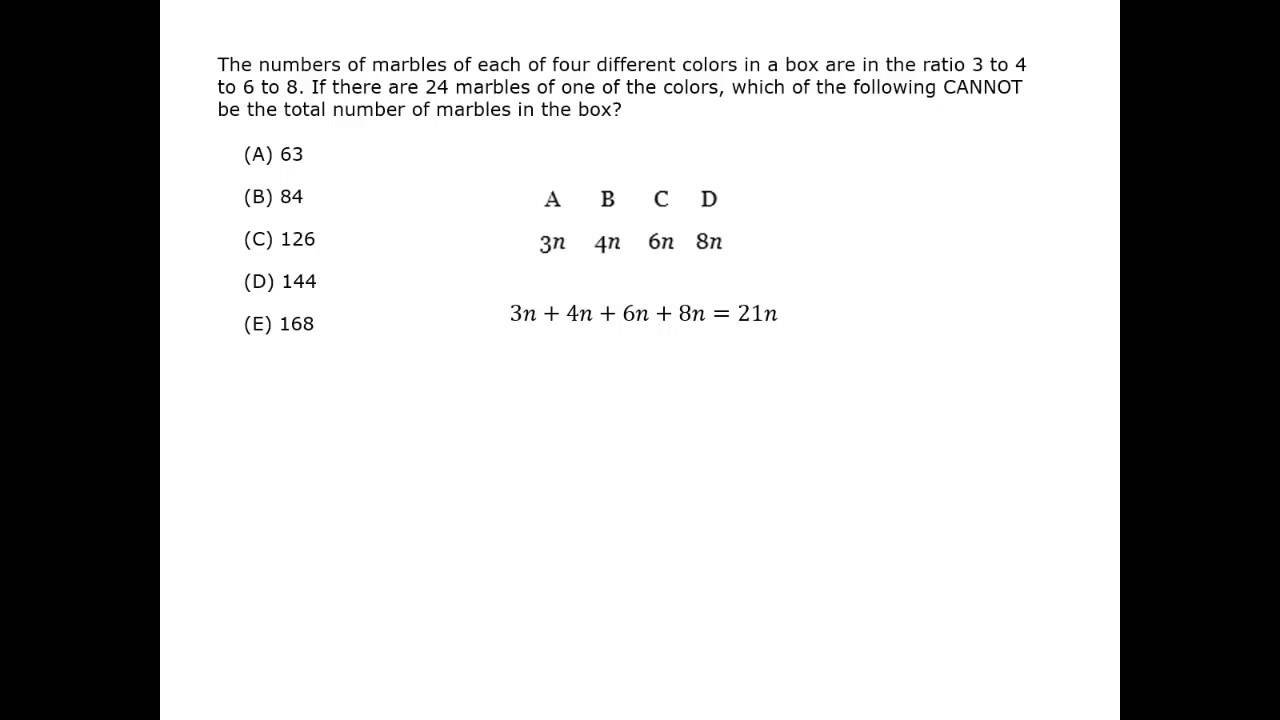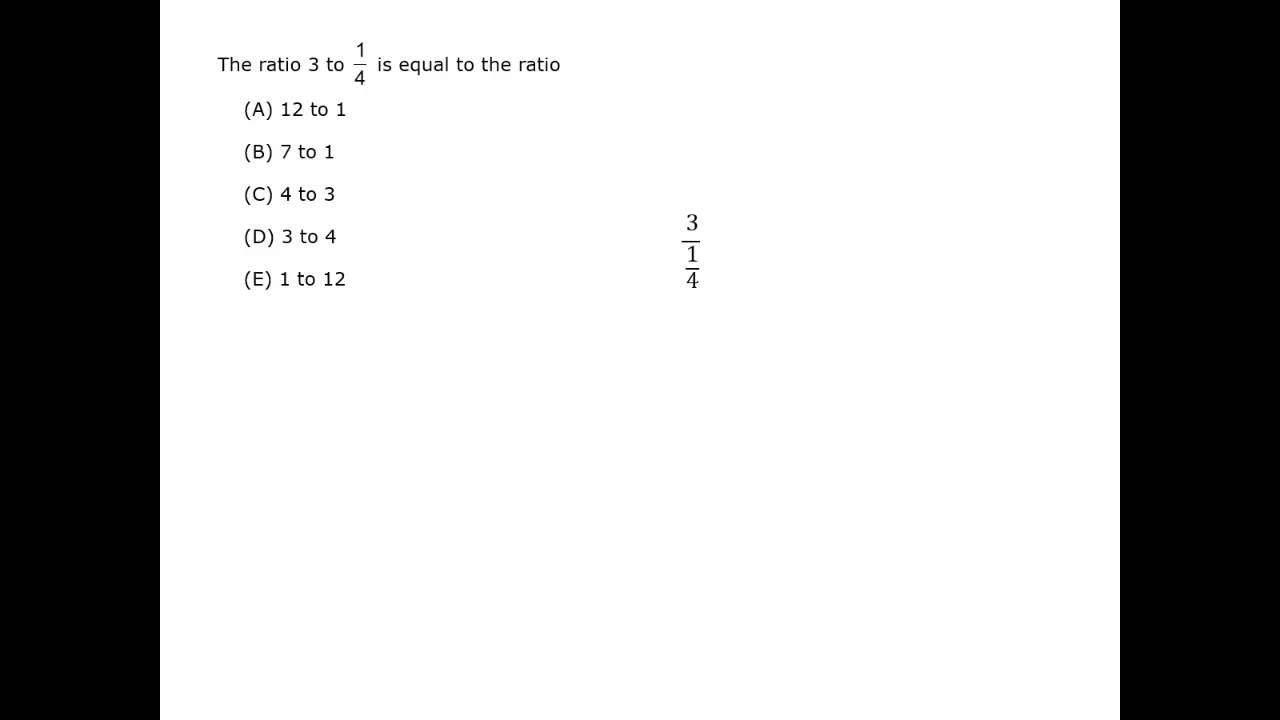#### Ratio

A ratio is used to compare two quantities.

The ratio of numbers a and b can be expressed as:

• the ratio of a to b
• a is to b
• a : b
• a/b

A fraction always compares a part to a whole. A ratio can compare a part to a part, or a part to a whole.

For example, if there are 2 oranges and 3 apples, the ratio of oranges to apples is 2:3.
The ratio and fraction of oranges to the total number of fruits is 2/5.

A baseball team won 98 out of the season’s 162 games.

(a) What is the ratio of wins to losses?

(b) What is the ratio of wins to games?

### Solution

(a) If the team won 98 games, they lost 64 games. So the ratio of wins to losses is 98 : 64, or 98/64.

(b) The ratio of wins to games is 98/162.

Orange concentrate is diluted with water to make juice that is 1/5 concentrate.

(a) What is the ratio of concentrate to water?

(b) What is the ratio of water to juice?

### Solution

(a) Juice that is 1/5 concentrate means that the total amount of juice is 5. One part of that total 5 is concentrate. The amount of water is total juice minus concentrate, so 5 – 1 = 4. The ratio of concentrate to water is 1 : 4.

(b) The fraction of concentrate in the total amount of juice is 1/5. Water makes up the rest of the juice, so its fraction is 4/5.

The ratio of two numbers is 4:1, and their sum is 40. Find the two numbers.

### Solution

Write the ratio of the two numbers x to y as x/y = 4/1 , so x = 4y

Their sum is 40, so x + y = 40.

4y + y = 40 Substitute x = 4y into x + y = 40
5y = 40
y = 8

x = 4y, so x = 32

The two numbers are 32 and 8.

Ratios can be between more than just two terms. One way to look at the relationship is that they are all multiples of one value.

The ratio is 1 : 2 : 5.
The total is 40.
So x + 2x + 5x = 40.

Red, blue and yellow marbles in a bag have a ratio of 5 to 2 to 6. After removing the red marbles, there are 32 marbles left in the bag. How many red marbles were in the bag?

(A) 4
(B) 8
(C) 20
(D) 24
(E) 52

### Solution

Use the total number of marbles in the bag and the ratio of just blue plus yellow.

blue + yellow = marbles left
2x + 6x = 32
8x = 32, so x = 4.

You can use x = 4 and the original 5 : 2 : 6 ratio to find the number of red marbles.
5x = 5 × 4 = 20
There were 20 red marbles in the bag.

The correct answer choice is (C).

Make sure you’re answering the question being asked. Here, the wrong answer options include x = 4, 2x = 8 blue marbles, 6x = 24 yellow marbles, and 32 + 20 = 52 marbles originally in the bag.

#### Ratio and Proportion

Best viewed in landscape mode

8 questions with video explanations

100 seconds per question#### Proportion

A proportion states that two ratios are equal.

A proportion comparing two ratios can be expressed as:

• a is to b as c is to d
• a : b = c : d
• a/b = c/d

You can solve for any term in a proportion: a, b, c or d.

Find the value of x. 35/84 = x/24

### Solution

Method 1

35 × 24 = 84x Cross multiply.

x = 35 × 24 /84 Divide both sides by 84.

x = 35 × 24/84 = 7 × 5 × 2 × 2 × 6/2 × 6 × 7 = 10 Factor and cancel to simplify the calculations.

x = 10

Method 2

35/84 = x/24 Factor and simplify the fractions.

7 × 5/7 × 12 = x/2 × 12
5/12 = x / (2 × 12)
5 = x / 2
x = 10

Find the value of x. 4 : 15 = 16 : x

### Solution

Write the proportion using fractions.

4/15 = 16/x

4x = 16 × 15 Cross multiply.

x = 16 × 15/4 = 4 × 15 = 60 Divide both sides by 4.
x = 60

If 100 dollars can buy 0.07 grams of a rare radioactive material, how many grams can you buy with 106 dollars?

(A) 7
(B) 70
(C) 700
(D) 7000
(E) 70,000

### Solution

Write the question as a proportion.

0.07/100 = g/106

0.07 × 106 = 100g Cross multiply.

g = (0.07 × 102 × 104)/100 = (7 × 104)/102= 7 × 102 Divide both sides by 100 and simplify.

g = 700

The correct answer choice is (C).

#### Inversely Proportional

Two quantities are directly proportional if one equals the other multiplied by a constant, or y = cx where c is a constant.
The Examples above were all directly proportional.

4/15 = 16/x is the same as 4 × 4/15 × 4 = 16/x

35/84 = x/24 is the same as 35/84 = 3.5x/3.5 × 24

When two quantities are directly proportional, both values increase or both values decrease.

Two quantities are inversely proportional if one decreases when the other increases.
One value is equal to a constant divided by the other, or:

y = c/x

This can be rewritten as:

xy = c

Here are some examples of inverse proportions:

• The unemployment rate is inversely proportional to economic growth. Unemployment goes up when economic growth goes down.
• The interest rate a company pays is inversely proportional to its credit rating. The better the credit rating, the lower the interest rate.
Roger drove 90 miles in 1.5 hours. How far would he go in 2.5 hours?

### Solution

This represents a direct proportion: both the distance and time increase.

90 miles/1.5 hours = m/2.5 hours

m = 90 × 2.5/1.5 = 90 × 5 × 0.5/3 × 0.5 = 30 × 5 = 150

Roger would drive 150 miles.

Roger drove 1 hour at 60 miles per hour. If he drove at 40 miles per hour, how long will it take to go the same distance?

### Solution

This represents an indirect proportion: as the speed decreases, the time increases.

60 mph × 1 hour = 40 mph × h hours
60 = 40h

h = 60/40 = 1.5

It will take 1.5 hours. The speed is 40/60 = 2/3 of the original speed, so the time is 3/2 = 1.5 times the original time.

It takes 4 men 6 hours to repair a road. Approximately how long will it take 7 men to do the job if they work at the same rate?

### Solution

This represents an indirect proportion: as the number of workers increases, the time decreases.

4 men × 6 hours = 1 job
7 men × h hours = 1 job

Set the 2 equations equal.

4 × 6 = 7h
h = 24/7 = 3/3/7 ≈ 3.5

It will take the 7 men about 3.5 hours.

#### Ratio and Proportion

Best viewed in landscape mode

7 questions with video explanations

100 seconds per question